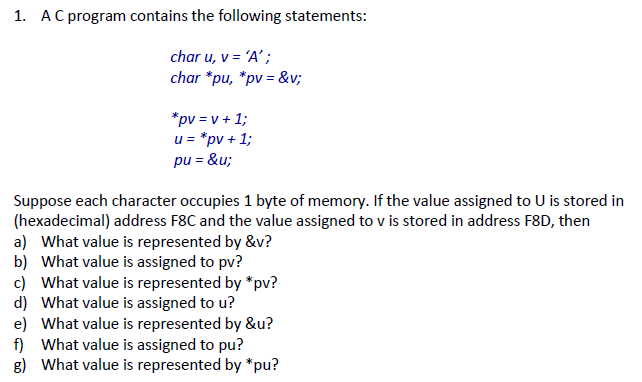# Question & Answer: AC program contains the following statements: char u, v = 'A': char *pu, *pv = &v: *pv = v + 1: u = *pv + 1: pu = &u: Suppose eac…..AC program contains the following statements: char u, v = ‘A’: char *pu, *pv = &v: *pv = v + 1: u = *pv + 1: pu = &u: Suppose each character occupies 1 byte of memory. If the value assigned to U is stored in (hexadecimal) address F8C and the value assigned to v is stored in address F8D, then a) What value is represented by &v? b) What value is assigned to pv? c) What value is represented by *pv? d) What value is assigned to u? e) What value is represented by &u? f) What value is assigned to pu? g) What value is represented by *pu?

Don't use plagiarized sources. Get Your Custom Essay on
Question & Answer: AC program contains the following statements: char u, v = 'A': char *pu, *pv = &v: *pv = v + 1: u = *pv + 1: pu = &u: Suppose eac…..
GET AN ESSAY WRITTEN FOR YOU FROM AS LOW AS \$13/PAGE

Solution:

It is not mentioned in the question that whether we need to answer if all the statements are executed, but I am considering all the statements are executed. If this is not the case please let me know in the comment section.

a)

The value represented by &v is F8D, because & is addressof operator which means the statement is asking the address of v.

b)

pv is a variable which contains the address of v and the value assigned to pv is F8D

c)

* is a dereference operator and the value represented by *pv is B, because at first ‘A’ is present at *pv (please note that pv containt &v and by * operator we are accessing what inside that address i.e. F8D)

but by the statement *pv= v+1;

it is increased by one hence the answer.

d)

The value assigned to u is ‘A’.

e)

The value assigned to &u is F8C.

f)

The value assigned to pu is F8C

g)

The value present at *pu is ‘C’, because first u=*pv+1 (‘B’+1) makes u to ‘C’ then the adress of u is assigned to pu.